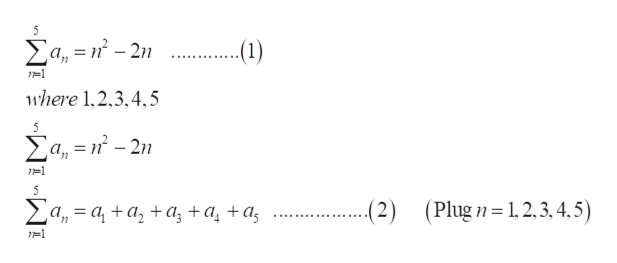# Given the sequence defined by an = n2 − 2n, find the fifth partial sum.

Question
17 views

Given the sequence defined by an = n2 − 2n, find the fifth partial sum.

check_circle

Step 1

To find the fifth partial sum from the given sequence of nth terms.

Step 2

Given information:

The sequence is shown below.

Step 3

Formula of 5th pa...help_outlineImage TranscriptioncloseΣα,--2η Ca, = n² – 2n .(1) =1 where 1,2,3,4,5 a, = n² – 2n E 1=1 (Plugn=1,2, 3, 4, 5) Za, = a, + a, +a; +a¸ +a; (2) 1=1 WI mWI fullscreen

### Want to see the full answer?

See Solution

#### Want to see this answer and more?

Solutions are written by subject experts who are available 24/7. Questions are typically answered within 1 hour.*

See Solution
*Response times may vary by subject and question.
Tagged in

### Algebra